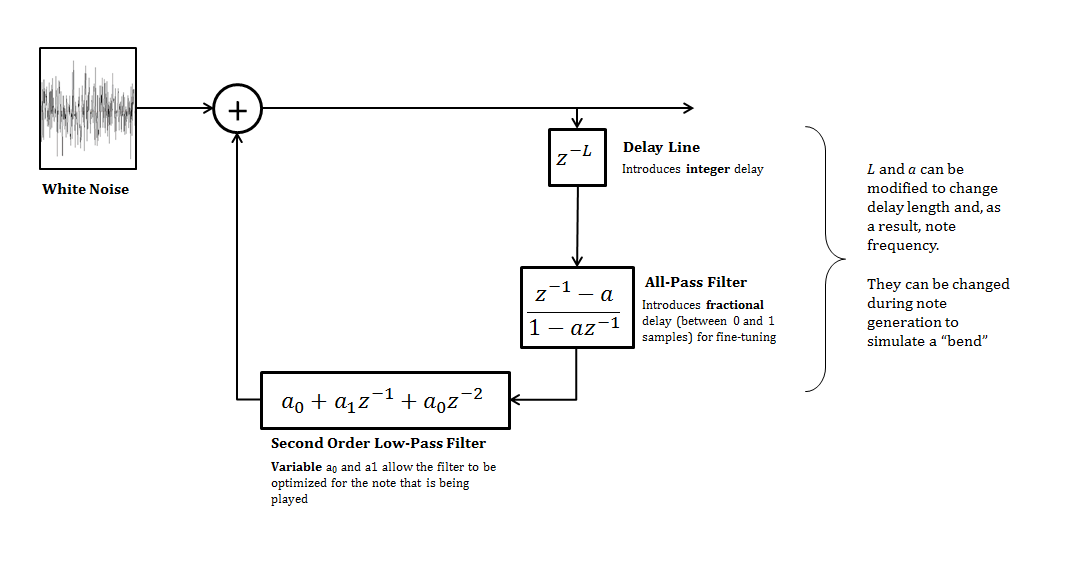# Comfortably Numb Solo Synthesis

During my last quarter at Rose-Hulman I took a great class called ECE481 - Electronic Music Synthesis. In it, we discussed a lot of concepts used to synthesize music, including additive and destructive synthesis, FM synthesis, the Karplus-Strong algorithm, and various post-processing and mixing techniques.

As a final project for the course we were asked to create a song using some of the techniques we learned about. For my project, I chose to synthesize the solo from the Pink Floyd song "Comfortably Numb" using the Karplus-Strong Algorithm.

To synthesize the guitar part of the song I wrote a modified version of the Karplus-Strong Algorithm - an algorithm for synthesizing the sound created by a plucked string. I made a few modifications to it to make the sound more realistic, which are described in detail below. Then I performed some post-processing on the guitar sound to make it sound like an electric guitar, including amplifier modeling, distortion and reverb. Finally, I used a basic MIDI file to synthesize the supporting instruments (drums, bass etc..).

I did the post-processing and instrument generation with a great, inexpensive Digital Audio Workstation (DAW) called Reaper.

## Modifications to Karplus-Strong

To get a basic understanding of the Karplus-Strong algorithm, you can read about it here.

I made some modifications to the basic Karplus-Strong algorithm for my project. Most of them were inspired by this page by Nicholas P. Donaldson.

My modifications included: - A variable second-order low-pass loop filter - An all-pass filter with adjustable delay to allow for continuous frequency selection - A dynamic delay line length and all-pass filter delay to allow for glissandi (bends)

My final K-S implementation (after all modifications) looked like this:## Low-Pass Filter

The original K-S implementation just uses a simple two-point averager for the Low-Pass filter, but I wanted something more configurable, so I used a second order filter as described by Donaldson:

$$H(z) = a_0 + a_1 z^{-1} + a_2 z^{-2}$$

A major problem this choice allowed me to solve is that of different notes decaying at different rates. Typically, K-S uses the same filter for every generated note. This means that higher notes get attenuated more by the low-pass filter and thus decay faster. This sounds very unrealistic, and I felt that it ruined my entire composition.

I was able to solve this problem by adusting the filter coefficients based on the frequency of the note being generated. I use the following design equations to compute these coefficients:

$$a_1 = \frac{0.999-\cos\left(2\pi\frac{4f_o}{f_s}\right)}{1-\cos\left(2\pi\frac{4f_o}{f_s}\right)} \text{, limited to } 0.5 \le a_1 \le 0.9$$
$$a_0 = \frac{1-a_1}{2}$$

Where $$f_o$$ is the note frequency and $$f_s$$ is the sampling frequency (usually 44.1 kHz).

These equations configure the filter so that it has a gain of 0.999 at 4 times the note frequency. That setting is arbitrary, but I found that it gave me a good amount of sustain over the entire range of notes that I was generating.

## All-Pass Filter

The original Karplus-Strong algorithm just used a delay line in the loop, but this only allows for a delay equal to an integer number of samples. To allow for finer delay adjustment, an all-pass filter is typically used. The all-pass filter introduces a fractional delay equal to $$1/a$$, which, when combined with the integer delay of the delay line, allows for a very wide range of continuous delay adjustment.

## Dynamic Delay

My implementation of the Karplus-Strong algorithm allows the delay line parameter, $$L$$, and the all-pass filter parameter, $$a$$, to be adjusted while the algorithm is running.

This dynamically adjusts the amount of delay in the loop, which adjusts the note frequency. Adjusting it during note generation creates a "bend".

## The Result

I used my custom algorithm to synthesize the entire guitar part of the solo. I used Reaper to do a little bit of post processing on the track and added a few other instruments. You can listen to the final result below: